# PySLM: Exposure Map Generation & Visualisation

A curious feature was seeing the possibility to both generate and visualise the exposure points generated in along scan vectors. This also includes generating a pseudo ‘heatmap’ or exposure map of the energy deposited by the laser scanning across the surface. Variations of this idea, I have seen across a variety of commercial software – in particular Renishaw’s QuantAM, which is perhaps the source of inspiration for coming up with an approach.

Usually, there is not much indication as the relative exposure of energy from the laser into a layer based both the chosen laser parameters applied to the scan vectors of a specific LayerGeometry group and also the overall spatial distribution of scan vectors in a region. For instance, overlaps between scan vectors will in theory deposit more energy across time into a localised region . Ideally, the exposure of energy from the laser is very uniform across the regions.

It is debatable whether there is particular usefulness of visualising the exposure map of the laser – but in practice can be used to differentiate laser scan parameters used on models in parametric studies. With further thought, a cheap numerical simulation could potentially capture the transient heat distribution generated by the sequential deposition of these exposure points.

#### A Light Background on Laser Exposure:

Hopefully someone can further comment on of this and perhaps correct me…

SLM systems are built using fiber laser systems offering a very fine, high quality controlled laser beam. Two types of laser operation exist: continuous wave (CW) and pulsed.

In practice, with pulsed lasers used in AM platforms, the scan vector is decomposed across a number of exposure points at a set ‘pointDistance’ from each other along the scan vector. Each exposure irradiates energy into the powder-bed surface at a fixed energy (inferred from the average laserPower) for a set time period referred as a ‘pointExposureTime‘. After exposure, the galvo-mirrors instantaneously move to direct the beam to the next exposure point. The relative speed this occurs optically gives the illusion that the laser is continuously moving. Continuous wave, as the name describes, emits continuous irradiation whilst the beam traverses the surface. The laser speed parameter is used here.

Different systems use either pulsed, continuous, or even both modes of operation, offering different benefits. This in principle is the reason for BuildStyle class containing the following parameters to cover both situations:

• Point Exposure Time
• Point Distance
• Laser Speed
• Laser Power – used across both modes

Ultimately, this does not matter, but the proposed method obviously requires the user to set at minimum the pointDistance parameter in order for the following methodology to work.

#### Proposed Methodology

Taking an existing Layer containing LayerGeometry, the exposure points are generated individually for each scan vector. Given the potential number of scan vectors within a layer, it would be beneficial to perform this efficiently.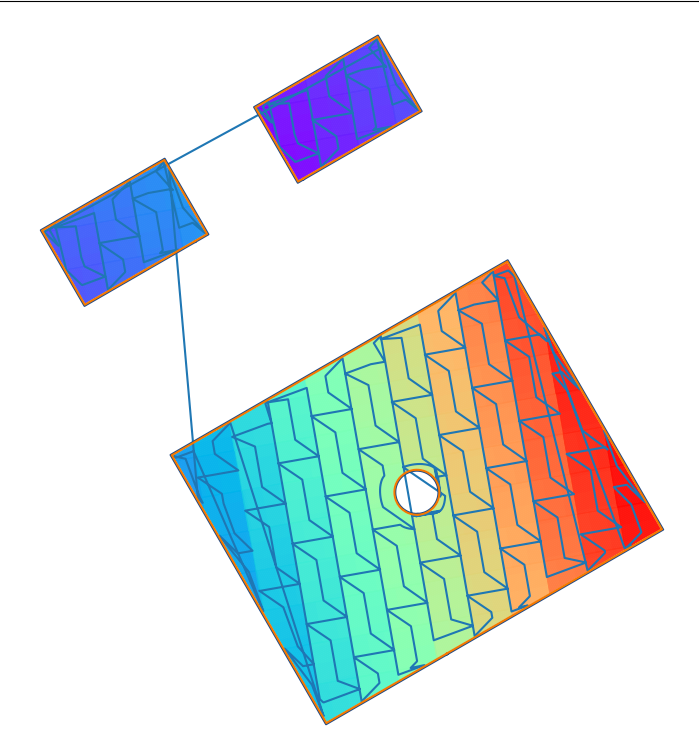Example part that is hatched with a series of overlapping 5mm island. The scan order is indicated by the blue line.

The basic process to do this is based on the following. The scan vector \textbf{v} has a particular length. Based on the point exposure distance, we can equally divide along this a number of points. Using both v_o and its direction ,we can then project a given number of exposure points along this line.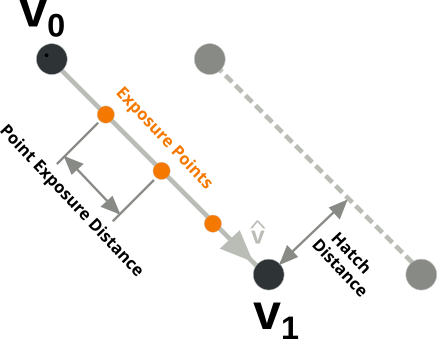Definition of a hath vector and decomposing each scan vector into a series of exposure points.

An attempt to do this more efficiently and exploit vectorisation built into numpy was achieved by the following procedure. The following properties of each scan vector (\textbf{v}) is obtained:

• start point (v_o)
• normalised scan vector direction (\hat{\textbf{v}})
• scan vector length \lVert \textbf{v} \rVert

For each LayerGeometry object, these are obtained across each scan vector as follows assuming that it is a HatchGeometry object:

# Calculate the length of the hatch vector and the direction
coords = layerGeom.coords.reshape(-1, 2, 2)
delta = np.diff(coords, axis=1).reshape(-1, 2)
lineDist = np.hypot(delta[:, 0], delta[:, 1]).reshape(-1, 1)

# Take the first coordinate
v0 = coords[:, 1, :].reshape(-1, 2)

# Normalise each scan vector direction
vhat = -1.0 * delta / lineDist

The number of point exposure across each vector is calculated by simple division. Unfortunately there is rounding, so the exposure may be missing at the end of the scan vector.

# Calculate the number of exposure points across the hatch vector based on its length
numPoints = np.ceil(lineDist / pointDistance).astype(np.int)


The total number of exposure points is calculated and is used to pre-populate some arrays, where the terms are used to extrapolate the exposure point distance (Line 16) are generated. Line 10 is key here and idxArray stores a range to account for each exposure point along the scan vector

Unfortunately, there isn’t a convenient way vectorise this so it is performed across each scan vector. Finally, the exposure points are generated by extrapolating them from v_o along \hat{\textbf{v}}.

# Pre-populate some arrays to extrapolate the exposure points from
totalPoints = int(np.sum(numPoints))
idxArray = np.zeros([totalPoints, 1])
pntsArray = np.zeros([totalPoints, 2])
dirArray = np.zeros([totalPoints, 2])

idx = 0
for i in range(len(numPoints)):
j = int(numPoints[i])
idxArray[idx:idx + j, 0] = np.arange(0, j)
pntsArray[idx:idx + j] = p0[i]
dirArray[idx:idx + j] = vhat[i]
idx += j

# Calculate the hatch exposure points
hatchExposurePoints = pntsArray + pointDistance * idxArray * dirArray

This generates a set of exposure points, which can be seen in the figure below:

Once the exposure points are generated, the deposited energy (laserPower x pointExposureTime) is added to each point and stored as a third column for later use.

#### Exposure Map Generation

Generating the exposure map is trivial. For a selected resolution chosen by the user, the bitmap slice is used currently to get the image to process with. The exposure coordinates are simply mapped, given an offset and resolution onto the image. The deposited energy is then summed accordingly across each pixel. The energy per area (latex]Q[/latex]), is calculated by diving by the aerial coverage of a single pixel.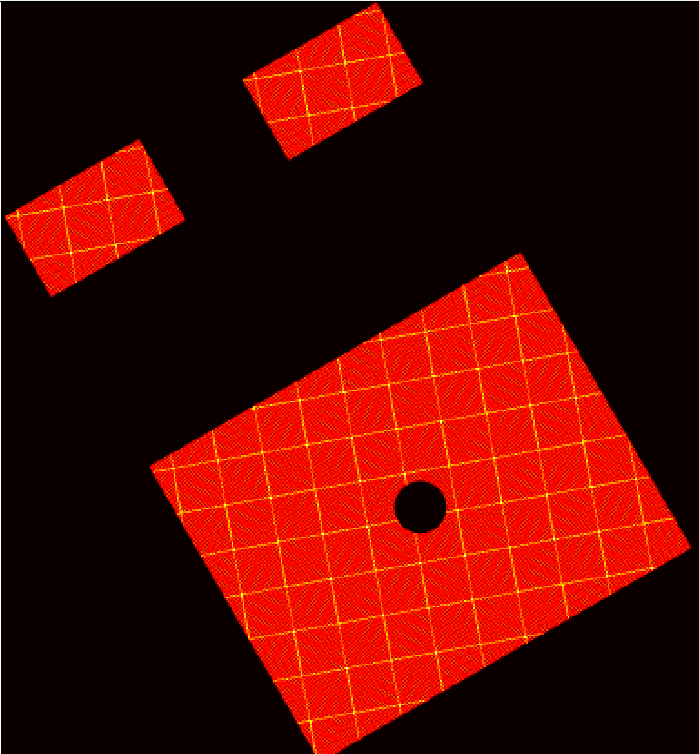Exposure / Heat Map showing the deposited energy applied to the position and laser parameters used. Notice the overlap region between islands show a higher energy deposition.

The resolution is arbitrarily selected. The above figure is generated with a resolution of 0.2 mm. It can be seen in this example that the there is a greater energy deposition across the the overlap regions between the islands. There are some ‘aliasing’ effects due to discretion onto a finer grid which is dependent on the resolution chosen.

## Conclusions

The above exposure map generation might have little scientific utility by itself. Rather it can offer a method to potentially visualise the energy deposition across a layer.

However, many thermo-mechanical AM build simulation that incorporate thermal effects in addition to the inherent strain approach could potentially incorporate a reference exposure map across the layer rather than assuming a single aerial heat-flux applied to the layer. This could actually be carried out across a ‘packet’ or number of layers to better correspond with the geometry and scan strategies employed.

## Documented Example

The code for this can be found in examples/example_heatmap.py on the github repository.

# Multi-threading Slicing & Hatching in PySLM

In PySLM, the slicing and hatching problem is inherently parallelisable simply because we can discretise areas the geometry into disrcete layers that for most situations behave independent. However, the actual underlying algorithms for slicing, offsetting the boundaries, clipping the hatch vectors is serial (single threaded). In order to significantly reduce the process time, multi-threaded processing is very desirable

Unfortunately, Python like most scripting or interpreter languages of the past are not inherently designed or destined to be multi-threaded. Perhaps, this may change in the future, but other scripting languages may fill this computational void (Rust, Julia, Go). Python, by intentions limits any multi-threaded use in scripts by using a construct known as the GIL – Global Interpreter Lock. This is a shared situation in other common scripting languages Matlab (ParPool), Javascript (Worker) where the parallel computing capability of multi-core CPUs cannot be exploited in a straightforward manner.

To some extent special distributions such as via the Anaconda distribution, core processing libraries such as numpy, scipy, scikit libraries internally are generally multi-threaded and support vectorisation via native CPU extensions. More computational mathematical operations and algorithms can to some extent be optimised to run in parallel automatically using numba, numexpr, and however, this cannot cover more broad multi-functional algorithms, such as those used in PySLM.

Python has the thread module for multi-threaded processing, however, for CPU bound processing it has very limited use This is because Python uses the global interpreter lock – GIL and this only allows one programming thread (i.e. one line) to be executed at any instance. It is mainly used for asynchronous IO (network or filesystem) operations which can be processed in the background.

#### Use of Multiprocessing Library in PySLM

The other option is to use the multiprocessing library built into the core Python distribution. Without going into too much of the formalities, multi-processing spawns multiple python processes and assign batches of work. The following programming article I found as a useful reference to the pitfalls of using the library.

In this implementation, the Pool and Manager modules are used to more optimally process the geometry. The most important section is to initialise the multiprocessing library with the ‘spawn‘ method, which stops random interruptions during the operation as discussed in the previous article.

from multiprocessing import Manager
from multiprocessing.pool import Pool
from multiprocessing import set_start_method

set_start_method("spawn")

The Manager.dict acts as a ‘proxy‘ object used to more efficiently store data which is shared between each process that is launched. Without using manager, for each process launch, a copy of the objects passed are made. This is important for the geometry or Part object, which if it were to contain a lattice of a set ofs complex surface would become expensive to copy.

d = Manager().dict()
d['part'] = solidPart
d['layerThickness'] = layerThickness # [mm]


A Pool object is used to create a set number of processes via setting the parameter processes=8 (typically one per CPU core). This is a fixed number re-used across a batch through the entire computation which removes the cost of additional copying and initialising many process instances. A series of z slice levels are created representing the layer z-id. These are then merged into a list of tuple pairs with the Manager dictionary and is stored in processList.

Pool.map is used to perform the slice function (calculateLayer) and collect all computed layers following the computation.

p = Pool(processes=8)

numLayers = solidPart.boundingBox / layerThickness
z = np.arange(0, numLayers).tolist()

processList = list(zip([d] * len(z), z))

# Run the pro
layers = p.map(calculateLayer, processList)

The slicing function is fairly straightforward and just unpacks the arguments and performs the slicing and hatching operation. Note: each layer needs to currently initialise its own instance of a Hatcher class because this is not shared across all the processes. This carries a small cost, but means each layer can process entirely independently; in this example the change is the hatchAngle across layers. The layer position is calculated using the layer position (zid) and layerThickness.

def calculateLayer(input):
# Typically the hatch angle is globally rotated per layer by usually 66.7 degrees per layer
d = input
zid= input

layerThickness = d['layerThickness']
solidPart = d['part']

# Create a StripeHatcher object for performing any hatching operations
myHatcher = hatching.Hatcher()

# Set the base hatching parameters which are generated within Hatcher
layerAngleOffset = 66.7
myHatcher.hatchAngle = 10 + zid * 66.7
myHatcher.volumeOffsetHatch = 0.08
myHatcher.spotCompensation = 0.06
myHatcher.numInnerContours = 2
myHatcher.numOuterContours = 1
myHatcher.hatchSortMethod = hatching.AlternateSort()

#myHatcher.hatchAngle += 10

# Slice the boundary
geomSlice = solidPart.getVectorSlice(zid*layerThickness)

# Hatch the boundary using myHatcher
layer = myHatcher.hatch(geomSlice)

# The layer height is set in integer increment of microns to ensure no rounding error during manufacturing
layer.z = int(zid*layerThickness * 1000)
layer.layerId = int(zid)

return zid

The final step to use multiprocessing in Python is the inclusion of the python __name__ guard i.e:

if __name__ == '__main__':
main()

The above is unfortunate because it makes debugging slightly more tedious in editors, but is the price for extra performance.

### Performance Improvement

The performance improvement using the multiprocesssing library is shown in the table below for a modest 4 core laptop (my budget doesn’t stretch that far).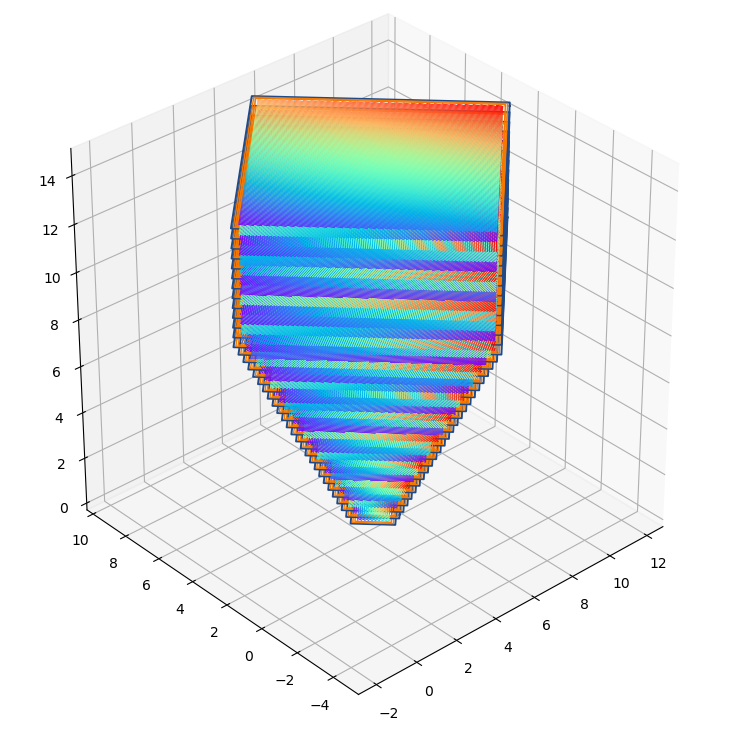Matplotlib Figure showing every subsequent 10 layers of hatching for the geometry but is shown reduced scale.

This was performed on the examples/inversePyramid.stl geometry with an overall bounding box size [90 \times 90 \times 60] mm, hatch distance h_d=0.08mm and the layer thickness set at 40 μm.

Given these are approximate timings, it is nearly linear performance improvement for the simple example. However, it can be seen choosing more processes beyond cores, does squeeze some extra performance out – perhaps due to Intel’s hyperthreading. Below shows that the CPU is fully utilised.

### Conclusions:

This post shows how one can adapt existing routines to generate multi-processing slicing and hatching with PySLM. In the future, it is desirable to explore a more integrated class structure for hooking functions onto. Other areas that are of interest to explore are potentially the use of GPU computing to parallelise some of the fundamental algorithms.

### Example

The example showing this that can be run is example_3d_multithread.py in the github repo.

# Improving Performance of Island Checkerboard Scan Strategy Hatching in PySLM

The hatching performance of PySLM using ClipperLib via PyClipper is reasonably good considering the age of the library using the Vatti polygon clipping algorithm. Without attempting to optimise the underlying library and clipping algorithm for most scenarios, the hatch clipping process should be sufficient for most use case. Future investigation will explore alternative clipping algorithms to further improve the performance of this intensive computational process

For the unfamiliar with the basic hatching process of a single layer, the laser or electron beam (a 1D single point source) must scan across an aerial (2D) region. This is done by creating a series of lines/vectors which infill or raster across the surface.

The most basic form of hatch infill for bulk regions is an alternating, meander, or in some locales referred to a serpentine scan strategy. This tends to be undesirable in SLM due to the creation of localised heat build-up resulting in porosity, poor surface finish , residual stress and resultant distortion and anisotropy due to preferential grain growth . Stripe or Island scan strategies are employed in attempt to mitigate these by limiting the length of scan vectors used across a region . Within the layer hatch vectors for each island are oriented orthogonal to each other and the scan vector length can be precisely controlled in order to reduce the magnitude of residual stresses generated .

However, when the user desires a stripe or an island scan strategy, the number of clipping operations for the individual hatch vectors increases drastically. The increase in number of clipping operations increases due to division of the area into fixed size regions corresponding to the desired scan vector length (typically 5 mm)]:

• Standard Meander Scan Strategy: n_{clip} \propto \frac{A}{hatchDistance(h_d)}
• Stripe Scan Strategy: n_{clip} \propto \frac{A}{StripeWidth}
• Island Scan Strategy: n_{clip} \propto \frac{A}{IslandWidth^2}

As can be observed, the performance of hatching with an island scan strategy degrades rapidly when using the island scan due to reciprocal square. As a result, using a naive approach, hatching a very large planar region using an island scan strategy could quickly result in 100,000+ clipping operations for a single layer for a large flat. In addition, this is irrespective of the sparsity of the layer geometry. The way the hatch filling approach works in PySLM, the maximum extent of a contour/polygon region is found. A circle is projected based on this maximum extent, and an outer bounding box is covered. This is explained in a previous post.

The scan vectors are tiled across the region. The reason behind this is to guarantee complete coverage irrespective of the chosen hatch angle, \theta_h, across the layer and largely simplifies the computation. The issue is that many regions will be outside the boundary of the part. Sparse regions both void and solid will not require additional clipping.

### The Proposed Technique:

In summary, the proposed technique takes advantage that each island is regular, and therefore each island can be used to discretise the region. This can be used to perform intersection tests for region that may be clipped, whilst recycling existing hatch vectors for those within the interior boundary.

Given that use an island scan strategy provides essentially structured grid, this can be easily transformed into a a method for selecting regions. Using the shapely library, each island boundary consisting of 4 edges can be quickly tested to check if it overlaps internally with the solid part and also intersected with the boundary. This is an efficient operation to perform, despite shapely (libGEOS) being not as efficient as PyClipper.

from shapely.geometry.polygon import LinearRing, Polygon

intersectIslands = []
overlapIslands = []

intersectIslandsSet = set()
overlapIslandsSet= set()

for i in range(len(islands)):

island = islands[i]
s = Polygon(LinearRing(island[:-1]))

if poly.overlaps(s):
overlapIslands.append(island)

if poly.intersects(s):
intersectIslands.append(island)

# Perform difference between the python sets
unTouchedIslandSet = intersectIslandsSet-overlapIslandsSet
unTouchedIslands = [islands[i] for i in unTouchedIslandSet]

This library is used because the user may re-test the same polygon consecutively, unlike re-building the polygon state in ClipperLib. Ultimately, this presents three unique cases:

1. Non-Intersecting (shapely.polygon.intersects(island) == False) – The Island resides outside of the boundary and is discarded,
2. Intersecting (shapely.polygon.intersects(island) == True) – The Island is in an internal region, but may be also clipped by the boundary,
3. Clipped (shapely.polygon.intersects(island) == True) – The island intersects with the boundary and requires clipping.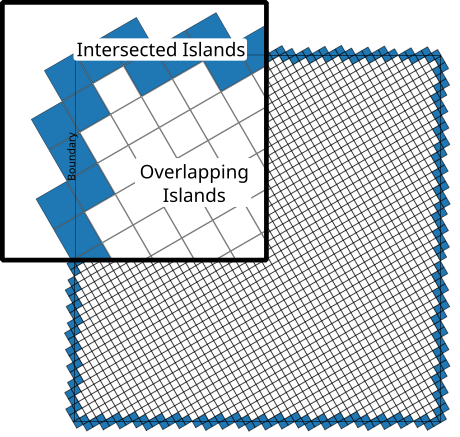The result is shown here for a simple 200 mm square filled with 5 mm islands:

Taking the difference between cases 2) and 3), the islands with hatch scan vectors can be generated without requiring unnecessary clipping of the interior scan vectors. As a result this significantly reduces the computational effort required.

Although extreme, the previous example generated a total number of 2209 5 mm islands to cover the entire region. The breakdown of the island intersections are:

1. Non-intersecting islands: 1591 (72%),
2. Non-clipped islands: 419 (19%),
3. Clipped islands: 199 (9%).

With respect to solid regions, the number of clipped islands account for 32% of the total area. The overall result is shown below. The total area of the hatch region that was hatched is 1.97 \times 10^3 \ mm^2, which is equivalent to a square length of 445 mm, significantly larger than what is capable on most commercial SLM systems. Using an island size of 5 mm with an 80 μm hatch spacing, the approximate hatching time is 6.5 s on a modest laptop system. For this example, 780 000 hatch vectors were generated.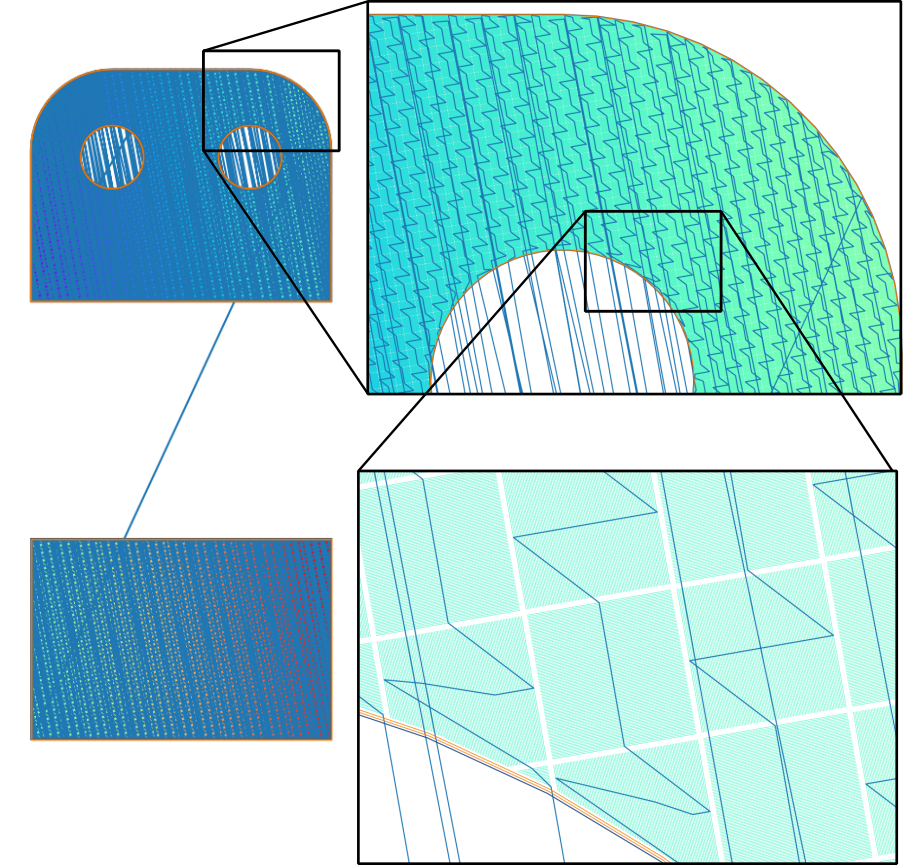A close up view showing the 5mm Island Hatching with 0.8 mm Hatch Distance. Blue Lines show the overall path traversed by the laser beam. The total time taken for hatching was approximately 8 seconds.

The order of hatching scanned is shown by the blue lines, which trace the midpoints of the vectors. Hatches inside the island are scanned sequentially. The order of scanning in this case is chosen to go vertically upwards and then horizontally across using the in-built Python 3 sorting function with a lambda expression Remarkably, all performed using one line:

sortedIslands = sorted(islands, key=lambda island: (island.posId, island.posId) )

A future post will elaborate further methods for sorting hatch vectors and island groups.

### Comparison to Original Implementation:

The following is a non-scientific benchmark performed to illustrate the performance profile of the proposed method in PySLM.

It is clearly evident that the proposed method reduces the overall time by 1-2 orders for hatching a region. What is strange is that with the new proposed method, the overall time increases with the island size.

Generally it is expected that the number of clipping operations n_{clip} to be the following:

n_{clip} \propto \frac{Perimiter}{IslandWidth}

Potentially, this allows bespoke complex ‘sub-island’ scan strategies to be employed without a significant additional cost because scan vectors within un-clipped island regions can be very quickly replicated across the layer.

### Other Benefits

The other benefits of taking approach is making a more modular object orientated approach for generating island based strategies, which don’t arbitrarily follow regular structured patterns. A future article will illustrate further explain the procedures for generating these.

The example can be seen and run in examples/example_island_hatcher.py in the Github repository.

References

↑1 Parry, L. A., Ashcroft, I. A., & Wildman, R. D. (2019). Geometrical effects on residual stress in selective laser melting. Additive Manufacturing, 25. https://doi.org/10.1016/j.addma.2018.09.026 Valente, E. H., Gundlach, C., Christiansen, T. L., & Somers, M. A. J. (2019). Effect of scanning strategy during selective laser melting on surface topography, porosity, and microstructure of additively manufactured Ti-6Al-4V. Applied Sciences (Switzerland), 9(24). https://doi.org/10.3390/app9245554 Zhang, W., Tong, M., & Harrison, N. M. (2020). Scanning strategies effect on temperature, residual stress and deformation by multi-laser beam powder bed fusion manufacturing. Additive Manufacturing, 36(June), 101507. https://doi.org/10.1016/j.addma.2020.101507 Ali, H., Ghadbeigi, H., & Mumtaz, K. (2018). Effect of scanning strategies on residual stress and mechanical properties of Selective Laser Melted Ti6Al4V. Materials Science and Engineering A, 712(October 2017), 175–187. https://doi.org/10.1016/j.msea.2017.11.103 Robinson, J., Ashton, I., Fox, P., Jones, E., & Sutcliffe, C. (2018). Determination of the effect of scan strategy on residual stress in laser powder bed fusion additive manufacturing. Additive Manufacturing, 23(February), 13–24. https://doi.org/10.1016/j.addma.2018.07.001 Mercelis, P., & Kruth, J.-P. (2006). Residual stresses in selective laser sintering and selective laser melting. Rapid Prototyping Journal, 12(5), 254–265. https://doi.org/10.1108/13552540610707013

# Build Time Estimation in L-PBF (SLM) Using PySLM (Part I)

Build-Time = Cost

This quantity is arguably the greatest driver of individual part cost for the majority of Additive Manufacture parts (excluding the additional costs of post-processing). It inherently relates to the proportional utilisation of the AM system that has a fixed capital cost at purchase under an assumed operation time (estimate is around 6-10 years).

Predicting this quickly and effectively for parts built using Powder Bed Fusion processes may initially sound simple, but actually there aren’t many free or opensource tools that provide a utility to predict this. Also the data isn’t not easily obtainable without having some inputs. In the literature, investigations into build-time estimation, embodied energy consumption and the analysis of costs associated with powder-bed for both SLM and EBM have been undertaken .

This usually involves submitting your design to an online portal or building up a spreadsheet and calculating some values. A large part of the cost for a part designed for AM is related to its build-time and this as a value can indicate the relative cost of the AM part.

Build-time, as a ‘lump’ measure is quintessentially the most significant factor in determining the ultimate cost of parts manufactured on powder-bed fusion systems. Obviously, this is oblivious to other factors such as post-processing of parts (i.e. heat-treatment, post-machining) surface coatings and post-inspection and part level qualification, usually essentially as part of the entire manufacturing processes for an AM part.

The reference to a ‘lump’ cost value coincides with various parameters inherent to the part that are driven by the decisions of design to meet the functional requirements / performance. The primary factors affecting this:

• Material alloy
• Geometrical shape of the part
• Machine system

These may be further specified as a set of chosen parameters

• Part Orientation
• Build Volume Packing (i.e. number of parts within the build)
• Number of laser beams in the SLM system
• Recoater time
• Material Alloy laser [arameters (i.e. effective laser scan speed)
• Part Volume (V)

From the build-time, the cost estimate solely for building the piece part can be calculated across ‘batches’ or a number of builds, which largely takes into account fixed costs such as capital investment in the machine and those direct costs associated with material inputs, consumables and energy consumption .

In this post, additional factors intrinsic to the machine operation, such as build-chamber warm-up and cool-down time, out-gassing time are ignored. Exploring the economics of the process, these should be accounted for because it can in some processes e.g. Selective Laser Sintering (SLS) and High-Speed-Sintering (HSS) of polymers can account for a significant contribution to the actual ‘accumulated‘ build time within the machine.

## Calculation of the Build Time in L-PBF

There are many different approaches for calculating the estimate of the build-time depending on the accuracy required.

### Build Bulk Volume Method

The build volume method is the most crudest forms for calculating the build time, t_{build}. The method takes the total volume of the part(s) within a build V and divided by machine’s build volume rate \dot{V} – a lumped empirical value corresponding to a specific material deposited or manufactured by an AM system.

t_{build}=\frac{V}{\dot{V}}

This is very approximate, therefore limited, because the prediction ignores build height within the chamber that is a primary contributor to the build time. Also it ignores build volume packaging – the density of numerous parts contained packed inside a chamber, which for each build contributes a fixed cost. However, it is a good measure for accounting the cost of the part based simply on its mass – potentially a useful indicator early during the design conceptualisation phase.

### Layer-wise Method

This approach accounts for the actual geometry of the part as part of the estimation. It performs slicing of the part and accounts for the area and boundaries of the part, which may be assigned separate laser scan speeds. This has been implemented as a multi-threaded/process example in order to demonstrate how one can analysis the cost of a part relatively quickly and simply using this as a template.

The entire part is sliced at the constant layer thickness L_t in the function calculateLayer(). In this function, the part is sliced using getVectorSlice(), at the particular z-height and by disabling returnCoordPaths parameter will return a list of Shapely.geometry.Polygon objects.

def calculateLayer(input):
d = input
zid= input

layerThickness = d['layerThickness']
solidPart = d['part']

# Slice the boundary
geomSlice = solidPart.getVectorSlice(zid*layerThickness, returnCoordPaths=False)

The slice represents boundaries across the layer. Each boundary is a Shapely.Polygon, which can be easily queried for its boundary length and area. This is performed later after the python multi-processing map call:

d = Manager().dict()
d['part'] = solidPart
d['layerThickness'] = layerThickness

# Rather than give the z position, we give a z index to calculate the z from.
numLayers = int(solidPart.boundingBox / layerThickness)
z = np.arange(0, numLayers).tolist()

# The layer id and manager shared dict are zipped into a list of tuple pairs
processList = list(zip([d] * len(z), z))

startTime = time.time()

layers = p.map(calculateLayer, processList)
p.close()
print('multiprocessing time', time.time()-startTime)

polys = []
for layer in layers:
for poly in layer:
polys.append(poly)

layers = polys

"""
Calculate total layer statistics:
"""
totalHeight = solidPart.boundingBox
totalVolume = solidPart.volume

totalPerimeter = np.sum([layer.length for layer in layers]) * numCountourOffsets
totalArea = np.sum([layer.area for layer in layers])

Once the sum of the total part area and perimeter are calculated the total scan time can be calculated from these. The approximate measure of scan time across the part volume (bulk region) is related by the total scan area accumulated across each layer of the partA, the hatch distance h_d and the laser scan speed v_{bulk}.

t_{hatch} = \frac{A}{L_t v_{bulk}}

Similarly the scan time across the boundary for contour scans (typically scanned at a lower speed is simply the total perimeter length L divided by the contour scan speed v_{contour}

t_{boundary} = \frac{L}{v_{contour}}

Finally, the re-coating time is simply a multiple of the number of layers.

"""
Calculate the time estimates
"""
hatchTimeEstimate = totalArea / hatchDistance / hatchLaserScanSpeed
boundaryTimeEstimate = totalPerimeter / contourLaserScanSpeed
scanTime = hatchTimeEstimate + boundaryTimeEstimate
recoaterTimeEstimate = numLayers * layerRecoatTime

totalTime = hatchTimeEstimate + boundaryTimeEstimate + recoaterTimeEstimate

### Compound approach using Surface and Volume

In fact, it may be possible to deduce that much of this is unnecessary for finding the approximate scanning time. Instead, a simpler formulation can be derived. The scan time can be deduced from simply the volume Vand the total surface area of the part S

t_{total}=\frac{V}{L_t h_d v_{bulk}} + \frac{S}{L_t v_{contour}} + N*t_{recoat},

where N=h_{build}/L_t. After realising this, further looking into literature, it was proposed by Giannatsis et al. back in 2001 for SLA time estimation . Surprisingly, I haven’t come across this before. They propose that taking the vertical projection of the surface better represents the true area of the boundary, under the slicing process.

t_{total}=\frac{V}{L_t h_d v_{bulk}} + \frac{S_P}{L_t v_{contour}} + N*t_{recoat}

The projected area is calculated by taking the dot product with the vertical vector v_{up} = (0.,0.,1.0)^T and the surface normal \hat{n} using the relation: a\cdot b = \|a\| \|b\| \cos(\theta) for each triangle and calculating the sine component using the identity (\cos^2(\theta) + \sin^2(\theta) = 1) to project the triangle area across the vertical extent.

""" Projected Area"""
# Calculate the vertical face angles
v0 = np.array([[0., 0., 1.0]])
v1 = solidPart.geometry.face_normals

sin_theta = np.sqrt((1-np.dot(v0, v1.T)**2))
triAreas = solidPart.geometry.area_faces *sin_theta
projectedArea = np.sum(triAreas)

## Comparison between build time estimation approaches

The difference in scan time with the approximation is relatively close for a simple example:

• Discretised Layer Scan Time – 4.996 hr
• Approximate Scan Time – 5.126 hr
• Approximate Scan Time (with projection) – 4.996 hr

Arriving at the rather simple result may not be interesting, but given the frequency of most cost models not stating this hopefully may be useful for some. It is useful in that it can account for the complexity of the boundary rather than simply the volume and the build-height, whilst factoring in the laser parameters used – typically available for most materials on commercial systems .

The second part of the posting will share more details about more precisely measuring the scan time using the analysis tools available in PySLM.

References

↑1 Baumers, M., Tuck, C., Wildman, R., Ashcroft, I., & Hague, R. (2017). Shape Complexity and Process Energy Consumption in Electron Beam Melting: A Case of Something for Nothing in Additive Manufacturing? Journal of Industrial Ecology, 21(S1), S157–S167. https://doi.org/10.1111/jiec.12397 Baumers, M., Dickens, P., Tuck, C., & Hague, R. (2016). The cost of additive manufacturing: Machine productivity, economies of scale and technology-push. Technological Forecasting and Social Change, 102, 193–201. https://doi.org/10.1016/j.techfore.2015.02.015 Faludi, J., Baumers, M., Maskery, I., & Hague, R. (2017). Environmental Impacts of Selective Laser Melting: Do Printer, Powder, Or Power Dominate? Journal of Industrial Ecology, 21(S1), S144–S156. https://doi.org/10.1111/jiec.12528 Liu, Z. Y., Li, C., Fang, X. Y., & Guo, Y. B. (2018). Energy Consumption in Additive Manufacturing of Metal Parts. Procedia Manufacturing, 26, 834–845. https://doi.org/10.1016/j.promfg.2018.07.104 Leach, R., & Carmignato, S. (2020). Precision Metal Additive Manufacturing (R. Leach & S. Carmignato. https://doi.org/10.1201/9780429436543 Giannatsis, J., Dedoussis, V., & Laios, L. (2001). A study of the build-time estimation problem for Stereolithography systems. Robotics and Computer-Integrated Manufacturing, 17(4), 295–304. https://doi.org/10.1016/S0736-5845(01)00007-2